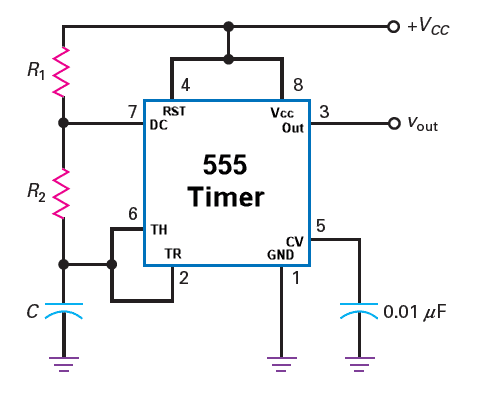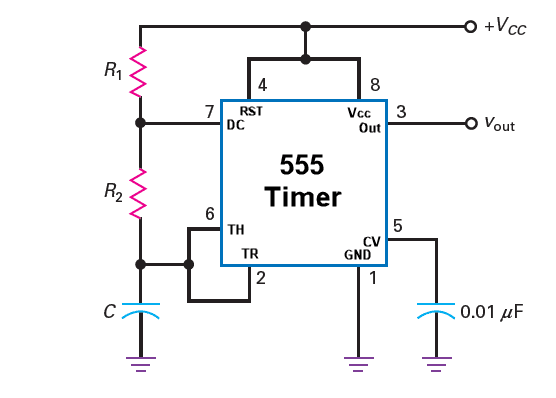# Mono Stable 555 Timer Calculator

Formula: $$t=1.1R_{}C_{}$$

Output:The above figure shows 555 timer circuit diagram for monostable operation. In monostable operation, the 555 timer produces a square wave of pulse width of certain time duration(t) when there is trigger input signal at the trigger pin 2. The resistor R and the capacitor C are used here to control the pulse width of pulse duration(t) and is given by the following formula. $t=1.1R_{}C_{}$ We can calculate the pulse width duration(t) using the above online 555 timer monostable calculator. For the trigger input we can connect a pulled high momentary push button to the trigger input pin 2. When we press the push button, there will be a square wave signal of width equal to the value obtained above.

# Astable Multivibrator 555 Timer Calculator

1) Calculate Frequency, Duty Cycle, Charging and Discharging Time:
Inputs:

Outputs:2) Calculate R1 from Frequency, R2 and C:
Inputs:

Outputs:

3) Calculate R1 from R2 and duty cycle:
Inputs:
%

Outputs:

Formula Used $$T_{H}=0.693(R_{1}+R_{2})C,\newline T_{L}=0.693R_{2}C,\newline f=\frac{1.44}{(R_{1}+2R_{2})C},\newline DC=\frac{R_{1}+R_{2}}{R_{1}+2R_{2}}$$